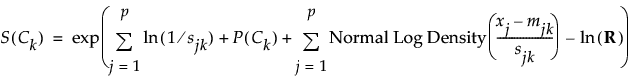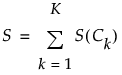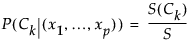Publication date: 04/12/2021

##Saved Probability Formulas

This section describes the formulas saved using the Save Probability Formula option. The conditional probability that an observation with predictor values x1, x2,, xp belongs in the class Ck differs slightly from that given by P(Ck|(x1,..., xp)), shown in the section Algorithm. This is done for computational efficiency.

#### Naive Score Formulas

The Naive Score formula for a given class Ck, S(Ck), is a variation of the numerator in the expression for P(Ck|(x1,..., xp)) and is computed as follows:#### Naive Score Sum Formulas

The Naive Score Sum formula, S, sums the Naive Score formulas over all classes. This is a variation of the denominator in the expression for P(Ck|(x1,..., xp)).#### Naive Prob Formulas

The Naive Prob formula for a given class Ck equals P(Ck|(x1,..., xp)). In the JMP formulas,#### Naive Predicted Formula

The Naive Predicted Formula for an observation classifies that observation into the class for which P(Ck|(x1,..., xp)) is the largest. This is equivalent to classifying an observation into the class for which its Naive Score formula is the largest.

Want more information? Have questions? Get answers in the JMP User Community (community.jmp.com).